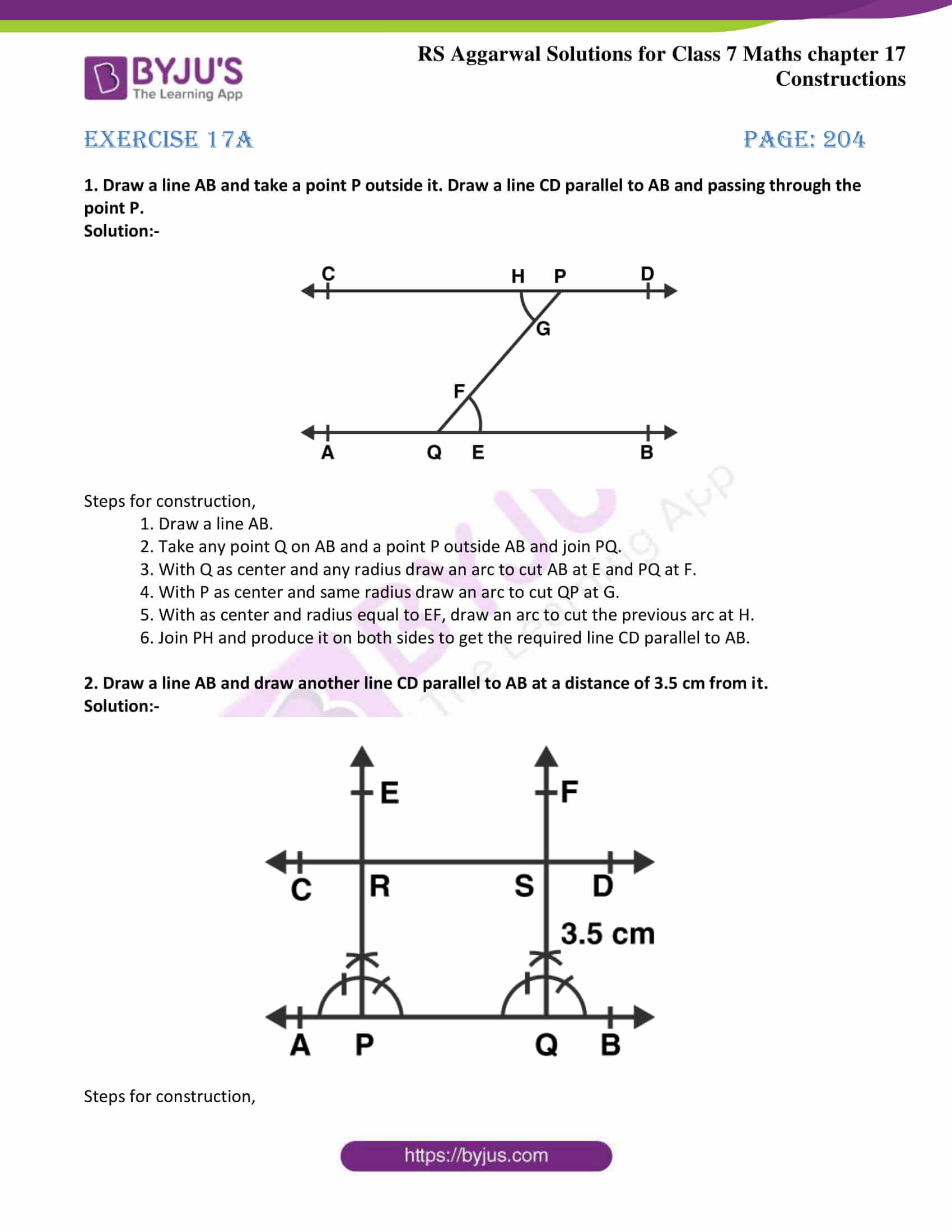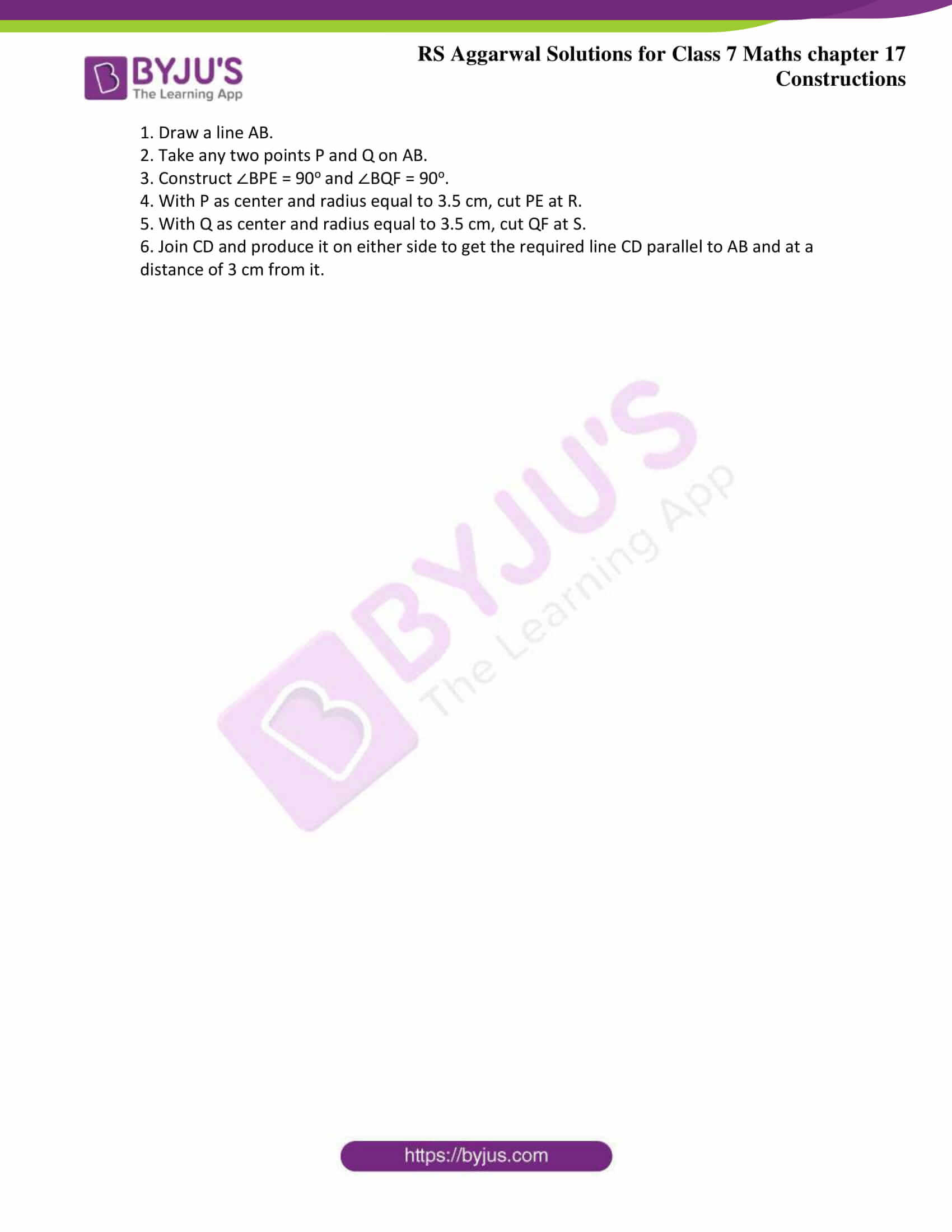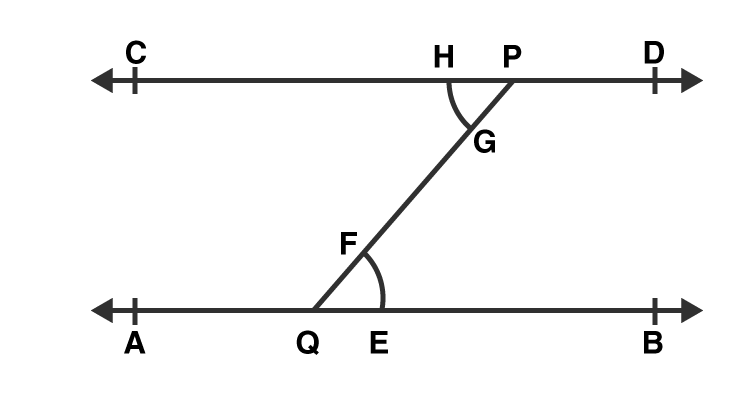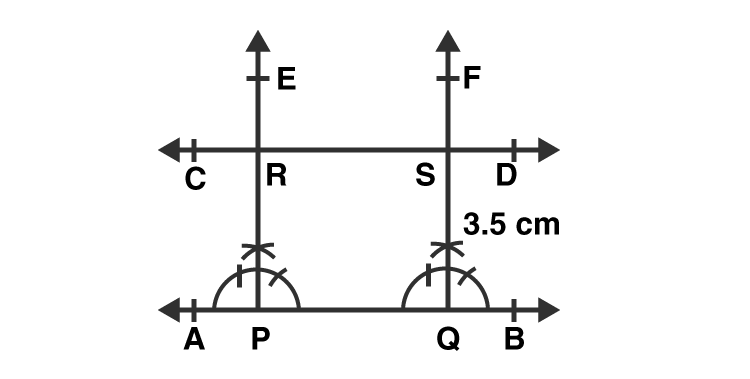# RS Aggarwal Solutions for Class 7 Maths Exercise 17A

RS Aggarwal Solutions for Class 7 Maths Exercise 17A Chapter 17 Constructions are given here in a simple and detailed way. RS Aggarwal Solutions for Class 7 Maths Chapter 17, solutions can be beneficial for the students to clear all their doubts quickly and understand the basics of this chapter. Students can use them as worksheets to prepare for their exams. The topics covered in this exercise are Introduction to Construction of a line parallel to a given line and construction of a line at a distance parallel to a given line. To download the PDF of RS Aggarwal Solutions for Class 7 Maths Chapter 17 Constructions, click on the link provided here.

## Download the PDF of RS Aggarwal Solutions For Class 7 Maths Chapter 17 Constructions – Exercise 17A### Access answers to Maths RS Aggarwal Solutions for Class 7 Chapter 17 – Constructions Exercise 17A

1. Draw a line AB and take a point P outside it. Draw a line CD parallel to AB and passing through the point P.

Solution:-Steps for construction,

1. Draw a line AB.

2. Take any point Q on AB and a point P outside AB and join PQ.

3. With Q as center and any radius draw an arc to cut AB at E and PQ at F.

4. With P as center and same radius draw an arc to cut QP at G.

5. With as center and radius equal to EF, draw an arc to cut the previous arc at H.

6. Join PH and produce it on both sides to get the required line CD parallel to AB.

2. Draw a line AB and draw another line CD parallel to AB at a distance of 3.5 cm from it.

Solution:-Steps for construction,

1. Draw a line AB.

2. Take any two points P and Q on AB.

3. Construct ∠BPE = 90o and ∠BQF = 90o.

4. With P as center and radius equal to 3.5 cm, cut PE at R.

5. With Q as center and radius equal to 3.5 cm, cut QF at S.

6. Join CD and produce it on either side to get the required line CD parallel to AB and at a distance of 3 cm from it.

### Access other exercises of RS Aggarwal Solutions For Class 7 Chapter 17 – Constructions

Exercise 17B Solutions

Exercise 17C Solutions